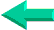Special

Excel Functions

2003-2010

## Lesson 18: HLOOKUP  Function In Excel

HLOOKUP allows to look for a value in a row based on a certain value in another row. So you look up for " John" in row 1 and you get his address in row 2.

HLOOKUP (working with rows)

IMPORTANT NOTE: The values within which you are looking up MUST BE IN ASCENDING ORDER (1, 2, 3, 4... or a, b, c, d). This is one of the reasons I switched to the magic function and INDEX/MATCH.

Here is a basic HLOOKUP formula: =HLOOKUP(2,A1:G32,4, FALSE)

The syntax for these formulas is  as follow, the first argument (2) is what you are looking for, the second argument (A1:G32) is the  range you are looking up within, the third argument (4) is the row from which the answer is extracted, and the fifth argument (FALSE) tells Excel that you are looking for an EXACT  match and not the next lower value. If you omit the fifth argument or use TRUE the formula will return the answer for the next lower value if it doesn't find EXACTLY what you are looking for. For example if you are looking up for Peter and the formula only finds Albert and Suzan it will return the answer for Albert. If you are looking up for 32 and Excel finds only 24 and 56 the formula will return the answer for 24.:
=HLOOKUP(2,A1:G32,4, FALSE) looks for " 2" in the first row (1) of the range (A1:G32) and returns the value of the cell in the 4th row (4) same column.

You can also use   the address of a cell in which you change the value as first argument in the formula (ex: J20)
=HLOOKUP(J20,A1:G32,4, FALSE) looks for whatever value is in cell J20 in the first row (1) of the range (A1:G32) and returns the value of the cell in the 4th row (4) same column.

Remember to ALWAYS use " FALSE" as fifth argument.

HLOOKUP is always looking up within the first row of the table that you submit as second argument. If you want the third row to be the look up column you need to change the second argument to a3:G32 and the third argument to 2.
=HLOOKUP(J20,C1:G32,2, FALSE)

HLOOKUP is a function inherited from Lotus-123. There is a more powerful and less limited way in Excel. It is the INDEX/MATCH formulas. INDEX/MATCH can replace all lookup functions (VLOOKUP, HLOOKUP and LOOKUP).Back home